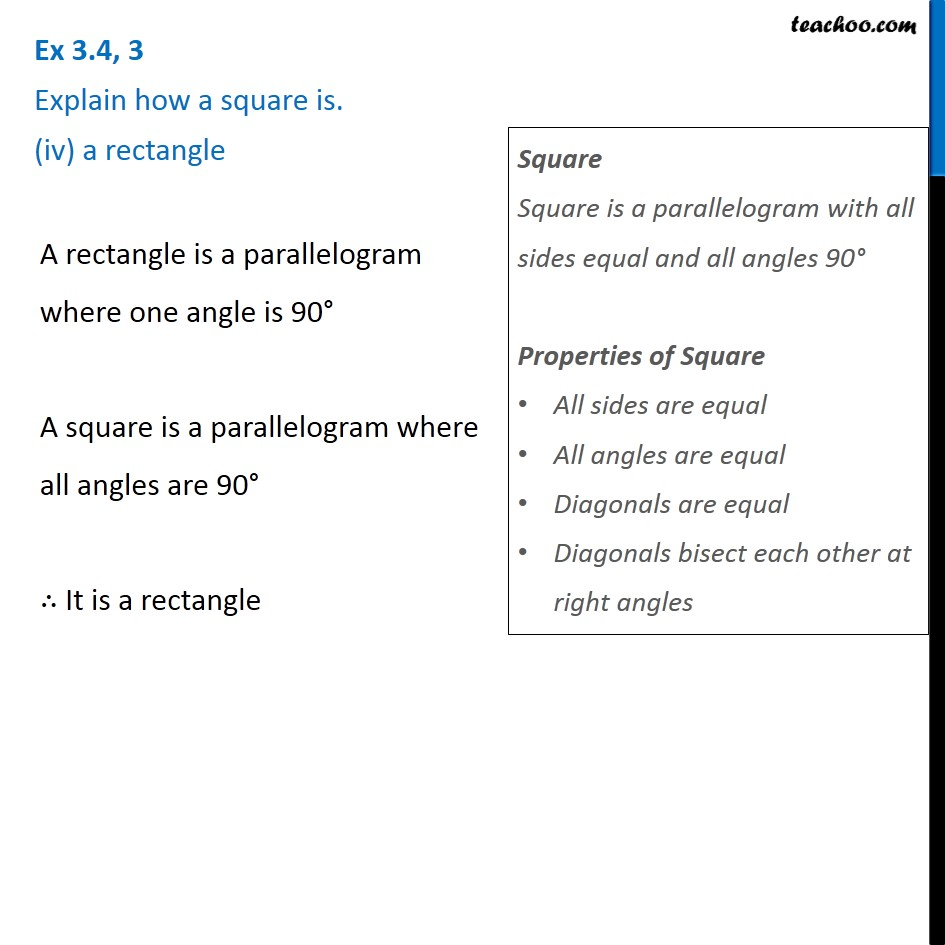1. Chapter 3 Class 8 Understanding Quadrilaterals
2. Serial order wise
3. Ex 3.4

Transcript

Ex 3.4, 3 Explain how a square is. (iv) a rectangle A rectangle is a parallelogram where one angle is 90° A square is a parallelogram where all angles are 90° ∴ It is a rectangle Square Square is a parallelogram with all sides equal and all angles 90° Properties of Square All sides are equal All angles are equal Diagonals are equal Diagonals bisect each other at right angles